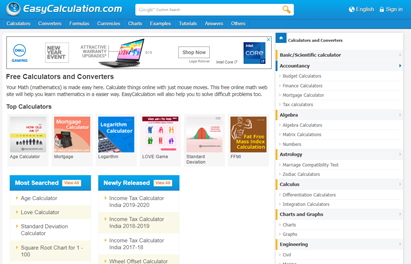www.easycalculation.com

# Easycalculation.com - FREE Online Math Calculator & Converter

Released On : 02-12-2005

Mathematics made easy through easycalculation.com. Calculate things online with just mouse moves. The site has FREE Online Math calculators, converters and tutorials. Use these simple math tools to solve complex calculations, conversions, logical and arithmetic problems. These free mathematics tools and tutorials help you obtain solutions in a simple way and learn mathematics easily.

Free mathematical calculation tools in this site include mathematics Calculater and Converters, Basic/ Scientific Calculater, Math Calci, Matrix Calculations, Hexa, Decimal, Binary Conversions, HEX Color Converter, Date and Day Calculator, Mortgage Calculator, Unit Conversions and Bandwidth Calculater.

Free online Mathematics Tutorials include topics on Trigonometry SIN, COS, TAN values, Arithmetic Mean, Median, Mode and Range, Standard Deviation, Geometric Mean, Prime numbers tutorial and Manual calculation, Fraction Basics and Manual conversions tutorial, Highest Common Factor and Least Common Multiple, Matrix Basic Definitions, Matrix Addition / Subtraction / Multiplication, Inverse of Matrix, Matrix Determinant, calculation of Bits, Bytes, Kibibytes, Kilobytes, etc.